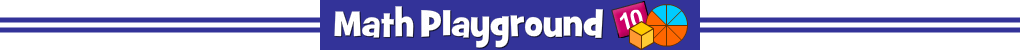Make a trail of numbers that add to the given sum.
Double click the final number to end the trail.
Number Trails Addition - Learning Connections
Essential Skills
Mental Math - practice adding numbers

Common Core Connection for Grades 1 and 2
Apply properties of operations as strategies to add and subtract.
Add and subtract within 20, demonstrating fluency for addition and subtraction within 10.
Add within 100, including adding a two-digit number and a one-digit number.
Fluently add and subtract within 20 using mental strategies.
More Math Games to Play